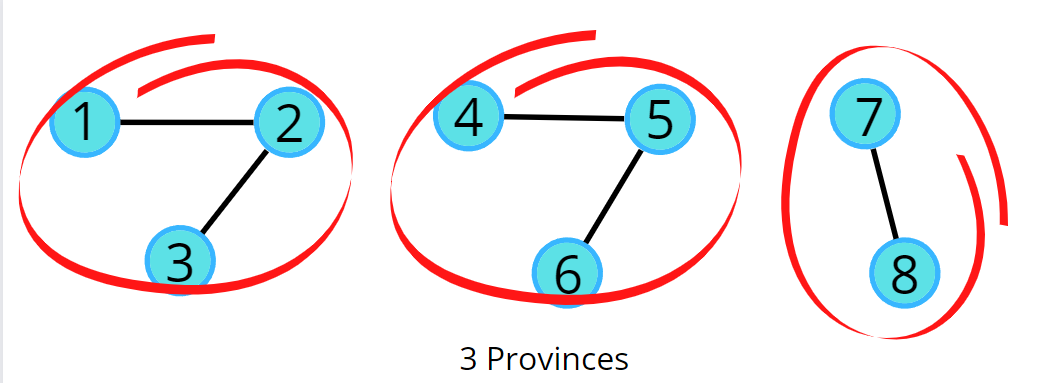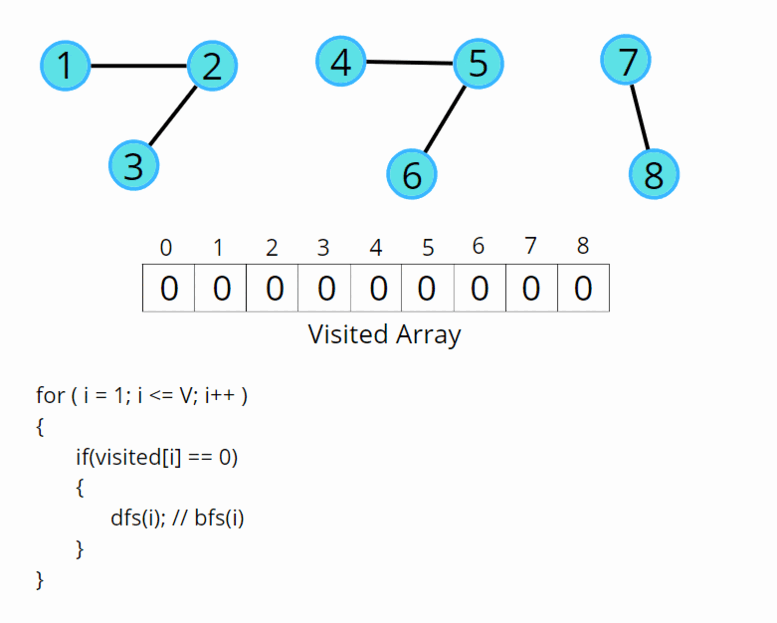# Number of Provinces

Problem Statement: Given an undirected graph with V vertices. We say two vertices u and v belong to a single province if there is a path from u to v or v to u. Your task is to find the number of provinces.

Pre-req: Connected Components, Graph traversal techniques

Examples:

```Input:Output: 3```

### Solution

Disclaimer: Don’t jump directly to the solution, try it out yourself first.

### Approach:

A province is a group of directly or indirectly connected cities and no other cities outside of the group. Considering the above example, we can go from 1 to 2 as well as to 3, from every other node in a province we can go to each other. As we cannot go from 2 to 4 so it is not a province. We know about both the traversals, Breadth First Search (BFS) and Depth First Search (DFS). We can use any of the traversals to solve this problem because a traversal algorithm visits all the nodes in a graph. In any traversal technique, we have one starting node and it traverses all the nodes in the graph. Suppose there is an ‘N’ number of provinces so we need to call the traversal algorithm ‘N‘ times, i.e., there will be ‘N’ starting nodes. So, we just need to figure out the number of starting nodes.

The algorithm steps are as follows:

• We need a visited array initialized to 0, representing the nodes that are not visited.
• Run the for loop looping from 0 to N, and call the DFS for the first unvisited node.
• DFS function call will make sure that it starts the DFS call from that unvisited node, and visits all the nodes that are in that province, and at the same time, it will also mark them as visited.
• Since the nodes traveled in a traversal will be marked as visited, they will no further be called for any further DFS traversal.
• Keep repeating these steps, for every node that you find unvisited, and visit the entire province.
• Add a counter variable to count the number of times the DFS function is called, as in this way we can count the total number of starting nodes, which will give us the number of provinces.Code:

## C++ Code

``````#include <bits/stdc++.h>
using namespace std;

class Solution {
private:
// dfs traversal function
void dfs(int node, vector<int> adjLs[], int vis[]) {
// mark the more as visited
vis[node] = 1;
for(auto it: adjLs[node]) {
if(!vis[it]) {
}
}
}
public:
int numProvinces(vector<vector<int>> adj, int V) {

// to change adjacency matrix to list
for(int i = 0;i<V;i++) {
for(int j = 0;j<V;j++) {
// self nodes are not considered
if(adj[i][j] == 1 && i != j) {
}
}
}
int vis[V] = {0};
int cnt = 0;
for(int i = 0;i<V;i++) {
// if the node is not visited
if(!vis[i]) {
// counter to count the number of provinces
cnt++;
}
}
return cnt;

}
};

int main() {

{
{1, 0, 1},
{0, 1, 0},
{1, 0, 1}
};

Solution ob;
cout << ob.numProvinces(adj,3) << endl;

return 0;
}
``````

Output: 2

Time Complexity: O(N) + O(V+2E), Where O(N) is for outer loop and inner loop runs in total a single DFS over entire graph, and we know DFS takes a time of O(V+2E).

Space Complexity: O(N) + O(N),Space for recursion stack space and visited array.

## Java Code

``````import java.util.*;

class Solution {
// dfs traversal function
private static void dfs(int node,
int vis[]) {
vis[node] = 1;
for(Integer it: adjLs.get(node)) {
if(vis[it] == 0) {
}
}
}
static int numProvinces(ArrayList<ArrayList<Integer>> adj, int V) {
ArrayList<ArrayList<Integer>> adjLs = new ArrayList<ArrayList<Integer>>();
for(int i = 0;i<V;i++) {
}

// to change adjacency matrix to list
for(int i = 0;i<V;i++) {
for(int j = 0;j<V;j++) {
// self nodes are not considered
if(adj.get(i).get(j) == 1 && i != j) {
}
}
}
int vis[] = new int[V];
int cnt = 0;
for(int i = 0;i<V;i++) {
if(vis[i] == 0) {
cnt++;
}
}
return cnt;
}
public static void main(String[] args)
{

ArrayList<ArrayList<Integer> > adj = new ArrayList<ArrayList<Integer> >();

Solution ob = new Solution();